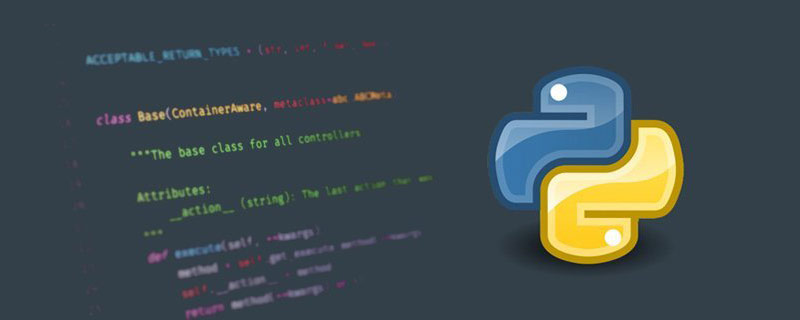• 导入所需的库
• 创建掩膜图像
• 方形掩膜
• 圆形掩膜
• 掩膜与原始图像进行拼接
• 展示图像
• 效果展示
• 总结

# 导入所需的库

```import cv2
import numpy as np复制代码```

# 创建掩膜图像

## 方形掩膜

```# 创建掩膜

## 圆形掩膜

x = 140
y = 100
r = 80

```# 创建掩膜
x = 140
y = 100
r = 80

# 掩膜与原始图像进行拼接

`image = cv2.add(img, np.zeros(np.shape(img), dtype=np.uint8), mask=mask)复制代码`

# 展示图像

```# 展示原图
cv2.imshow("img", img)
# 展示掩膜图片
# 展示添加掩膜效果图片
cv2.imshow("image", image)复制代码```

# 总结

## VSCode中怎么配置Python，实现错误提醒和自动格式化

VSCode中怎么配置Python，实现错误提醒和自动格式化

## python如何随机读取目录文件_亿码酷站_亿码酷站

python如何随机读取目录文件_亿码酷站_亿码酷站